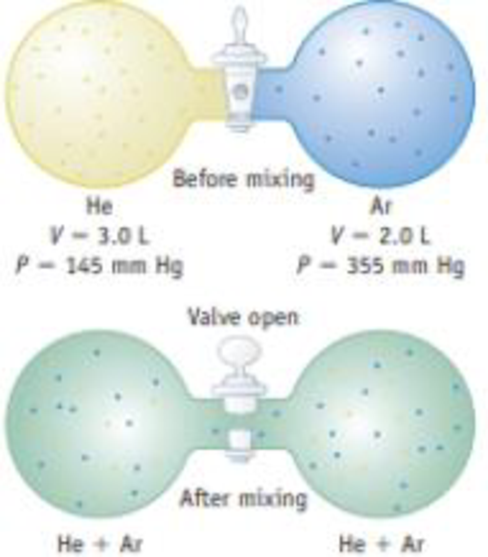# A 3.0-L bulb containing He at 145 mm Hg is connected by a valve to a 2.0-L bulb containing Ar at 355 mm Hg (see Figure). Calculate the partial pressure of each gas and the total pressure after the valve between the flasks is opened.### Chemistry & Chemical Reactivity

9th Edition
John C. Kotz + 3 others
Publisher: Cengage Learning
ISBN: 9781133949640

#### Solutions

Chapter
Section### Chemistry & Chemical Reactivity

9th Edition
John C. Kotz + 3 others
Publisher: Cengage Learning
ISBN: 9781133949640
Chapter 10, Problem 76GQ
Textbook Problem
241 views

## A 3.0-L bulb containing He at 145 mm Hg is connected by a valve to a 2.0-L bulb containing Ar at 355 mm Hg (see Figure). Calculate the partial pressure of each gas and the total pressure after the valve between the flasks is opened.Interpretation Introduction

Interpretation: For the two given set of gases each with given volume and pressure the partial pressure of each gas and the total pressure of the gas after valve has to be opened.

Concept introduction:

Ideal gas Equation:

Any gas can be described by using four terms namely pressure, volume, temperature and the amount of gas.  Thus combining three laws namely Boyle’s, Charles’s Law and Avogadro’s Hypothesis the following equation could be obtained.  It is referred as ideal gas equation.

nTPV = RnTPPV = nRTwhere,n = moles of gasP = pressureT = temperatureR = gas constant

Under some conditions gases don not behave like ideal gas that is they deviate from their ideal gas properties.  At lower temperature and at high pressures the gas tends to deviate and behave like real gases.

Boyle’s Law:

At given constant temperature conditions the mass of given ideal gas in inversely proportional to the volume.

Charles’s Law:

At given constant pressure conditions the volume of ideal gas is directly proportional to the absolute temperature.

Two equal volumes of gases with same temperature and pressure conditions tend to have same number of molecules with it.

Van der Waal’s gas equation:

The van der Waal equation describes the ideal gas as it approaches to zero.  The van der Waal equation contains correction terms a and b for the intermolecular forces and molecular size respectively.

The van der Waal equation is as follows,

[P+a(nV)2](Vnb)=RT

### Explanation of Solution

Given,

Before mixingHeArV 3.0 L2.0 LP145 mm Hg

### Still sussing out bartleby?

Check out a sample textbook solution.

See a sample solution

#### The Solution to Your Study Problems

Bartleby provides explanations to thousands of textbook problems written by our experts, many with advanced degrees!

Get Started

Find more solutions based on key concepts
An example of a person in positive nitrogen balance is a pregnant woman. T F

Nutrition: Concepts and Controversies - Standalone book (MindTap Course List)

Match the blood terms with the best description.

Human Biology (MindTap Course List)

What is the difference between artificial selection and natural selection?

Biology: The Dynamic Science (MindTap Course List)

Assign an IUPAC name to each of the following alcohols.

General, Organic, and Biological Chemistry

Has anybody drilled into Earths mantle or core to return samples of the densest interior layers?

Oceanography: An Invitation To Marine Science, Loose-leaf Versin

Figure P2.43 represents part of the performance data of a car owned by a proud physics student, (a) Calculate t...

Physics for Scientists and Engineers, Technology Update (No access codes included)